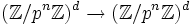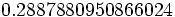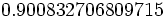# Cohen-Lenstra measure

## Definition

Let$p$ be a prime number. The Cohen-Lenstra measure is a probability measure on the set of isomorphism classes of finite abelian$p$-groups (i.e., the set of abelian groups whose order is a power of the prime$p$), where the measure value on a particular isomorphism class of abelian$p$-group$P$ is:$\frac{1}{\left|\operatorname{Aut}(P)\right|} \prod_{j=1}^\infty \left( 1 - \frac{1}{p^j} \right)$

The Cohen-Lenstra measure value for a group$P$ can be defined as the limit as$n \to \infty, d \to \infty$ of the probability that the kernel of the map:$(\mathbb{Z}/p^n\mathbb{Z})^d \to (\mathbb{Z}/p^n\mathbb{Z})^d$

is isomorphic to$P$, where probability here simply means the quotient of the number of homomorphisms$(\mathbb{Z}/p^n\mathbb{Z})^d \to (\mathbb{Z}/p^n\mathbb{Z})^d$ with kernel isomorphic to$P$ to the total number of homomorphisms$(\mathbb{Z}/p^n\mathbb{Z})^d \to (\mathbb{Z}/p^n\mathbb{Z})^d$.

### Equivalence of definitions

Further information: equivalence of definitions of Cohen-Lenstra measure

## Particular cases

### Value of the product

The product$\prod_{j=1}^\infty \left( 1 - \frac{1}{p^j} \right)$ is the$q$-Pocchammer symbol with parameters$1/p$ and$1/p$. The approximate values are given below:$p$$\prod_{j=1}^\infty \left( 1 - \frac{1}{p^j} \right)$ Its reciprocal (equal to$\sum 1/|\operatorname{Aut}(P)|$)
2$\! 0.2887880950866024$$\! 3.462746619455064$
3$\! 0.5601260779279490$$\! 1.785312341998534$
5$\! 0.7603327958712324$$\! 1.315213555735345$
7$\! 0.8367954070890379$$\! 1.195035239830847$
11$\! 0.900832706809715$$\! 1.110084028300309$
13$\! 0.917162472540942$$\! 1.090319359916201$
17$\! 0.937716969709066$$\! 1.066419860472673$
19$\! 0.944598742929940$$\! 1.058650572515285$
23$\! 0.954631535623623$$\! 1.047524581666726$

For the prime$p = 2$, we list the Cohen-Lenstra measures of some small abelian$p$-groups:$P$ prime-base logarithm of order partition corresponding to decomposition as product of cyclic groups order of automorphism group Cohen-Lenstra measure
trivial group 0 empty 1$\! 0.288788$
cyclic group:Z2 1 1 1$\! 0.288788$
cyclic group:Z4 2 2 2$\! 0.144394$
Klein four-group 2 1 + 1 6$\! 0.0481313$
cyclic group:Z8 3 3 4$\! 0.072197$
direct product of Z4 and Z2 3 2 + 1 8$\! 0.0360985$
elementary abelian group:E8 3 1 + 1 + 1 168$\! 0.00171898$